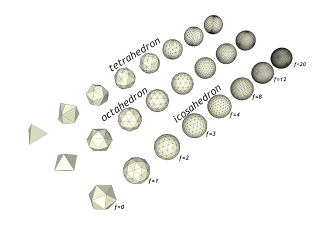## giovedì 29 dicembre 2011

### Geodesic dome calculation in RubyProblem: Given the faces for a tetrahedron, octahedron, or isocahedron, create a geodesic dome of arbitrary frequency. Note: The (equilateral triangle) faces of each primitive are given as triplets of vertex points. Each vertex is itself a triplet of cartesian 3-space coordinates, all of unit-distance from 0,0,0. (See the supplied points at the end for an example.) The resulting geodesic should be an array of triangular faces; again each face is a triplet of points, and each point is unit-distance from 0,0,0. Solution: due in 30 minutes of Ruby programming! ù